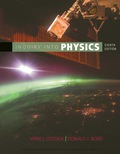# . The frequency of the highest note on the piano is 4.186 Hz. (a) How many harmonics of that note can we hear? (b) How many harmonics of the note one octave below it can we hear?### Inquiry into Physics

8th Edition
Ostdiek
Publisher: Cengage
ISBN: 9781337515863

#### Solutions

Chapter
Section### Inquiry into Physics

8th Edition
Ostdiek
Publisher: Cengage
ISBN: 9781337515863
Chapter 6, Problem 26P
Textbook Problem
1 views

## . The frequency of the highest note on the piano is 4.186 Hz. (a) How many harmonics of that note can we hear? (b) How many harmonics of the note one octave below it can we hear?

To determine

(a)

The number of notes which can we hear.

### Explanation of Solution

Given:

• The frequency of highest note is 4186Hz.

Formula used:

The frequency of the nth harmonic is n times the frequency of the first harmonic.

So, formula for nth harmonic is,

fn=nf1

Here, fn is the frequency of nth harmonic and f1 is the frequency of first harmonic.

Calculation:

Find the frequency of first a few note which comes in the audible range.

The first note:

f1=4186Hz

The second note:

f2=2×4186Hz=8372Hz

To determine

(b)

The number of harmonic notes can we hear.

### Still sussing out bartleby?

Check out a sample textbook solution.

See a sample solution

#### The Solution to Your Study Problems

Bartleby provides explanations to thousands of textbook problems written by our experts, many with advanced degrees!

Get Started

Find more solutions based on key concepts
LDL, a class of lipoprotein, delivers triglycerides and cholesterol from the liver to the bodys tissues. T F

Nutrition: Concepts and Controversies - Standalone book (MindTap Course List)

How many centimeters are there in a kilometer?

An Introduction to Physical Science

Match the terms and definitions.

Human Biology (MindTap Course List)

How can errors in the cell cycle lead to cancer in humans?

Human Heredity: Principles and Issues (MindTap Course List)

24. Name each compound:

Chemistry In Focus

Two point charges attract each other with an electric force of magnitude F. If the charge on one of the particl...

Physics for Scientists and Engineers, Technology Update (No access codes included)

How can wind-driven horizontal movement of water induce vertical movement in surface water?

Oceanography: An Invitation To Marine Science, Loose-leaf Versin